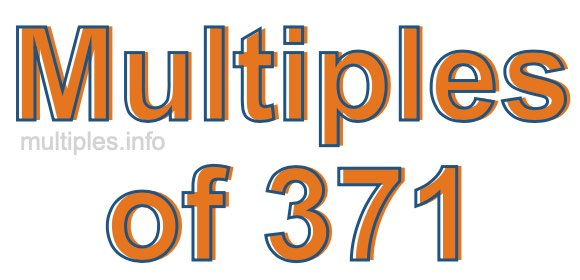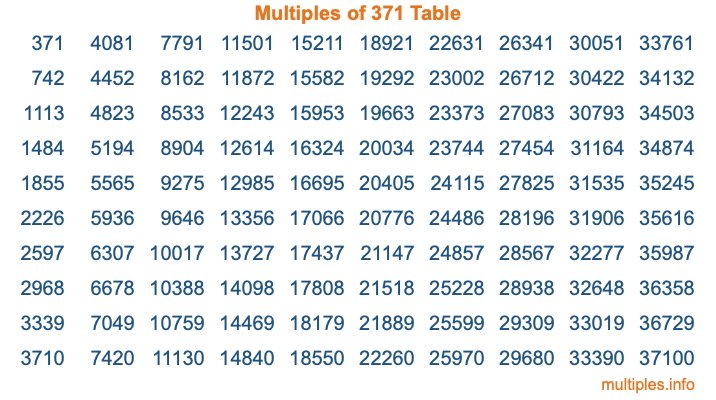Multiples of 371Welcome to the Multiples of 371 page. Here we will first teach you everything you will ever need to know about the multiples of 371, and then give you a study guide summary of everything we taught you to make sure you remember it all. Use this page to look up facts and learn information about the multiples of 371. This page will make you a multiples of three hundred seventy-one expert!

Definition of Multiples of 371
Multiples of 371 are all the numbers that when divided by 371 equal an integer. Each of the multiples of 371 are called a multiple. A multiple of 371 is created by multiplying 371 by an integer.

Therefore, to create a list of multiples of 371, you start with 1 multiplied by 371, then 2 multiplied by 371, then 3 multiplied by 371, and so on for as long as you want. Thus, the list of the first five multiples of 371 is 371, 742, 1113, 1484, and 1855. To see a larger list of multiples of 371, see the printable image of Multiples of 371 further down on this page. We also have a category where you can choose any nth multiple of 371.

Multiples of 371 Checker
The Multiples of 371 Checker below checks to see if any number of your choice is a multiple of 371. In other words, it checks to see if there is any number (integer) that when multiplied by 371 will equal your number. To do that, we divide your number by 371. If the the quotient is an integer, then your number is a multiple of 371.

Is  a multiple of 371?

Least Common Multiple of 371 and ...
A Least Common Multiple (LCM) is the lowest multiple that two or more numbers have in common. This is also called the smallest common multiple or lowest common multiple and is useful to know when you are adding our subtracting fractions. Enter one or more numbers below (371 is already entered) to find the LCM.

Check out our LCM Calculator if you need more details about the Least Common Multiple or if you need the LCM for different numbers for adding and subtraction fractions.

nth Multiple of 371
As we stated above, 371 is the first multiple of 371, 742 is the second multiple of 371, 1113 is the third multiple of 371, and so on. Enter a number below to find the nth multiple of 371.

th multiple of 371

Multiples of 371 vs Factors of 371
371 is a multiple of 371 and a factor of 371, but that is where the similarities end. All postive multiples of 371 are 371 or greater than 371. All positive factors of 371 are 371 or less than 371.

Below is the beginning list of multiples of 371 and the factors of 371 so you can compare:

Multiples of 371: 371, 742, 1113, 1484, 1855, etc.

Factors of 371: 1, 7, 53, 371

As you can see, the multiples of 371 are all the numbers that you can divide by 371 to get a whole number. The factors of 371, on the other hand, are all the whole numbers that you can multiply by another whole number to get 371.

It's also interesting to note that if a number (x) is a factor of 371, then 371 will also be a multiple of that number (x).

Multiples of 371 vs Divisors of 371
The divisors of 371 are all the integers that 371 can be divided by evenly. Below is a list of the divisors of 371.

Divisors of 371: 1, 7, 53, 371

The interesting thing to note here is that if you take any multiple of 371 and divide it by a divisor of 371, you will see that the quotient is an integer.

Multiples of 371 Table
Below is an image of the first 100 multiples of 371 in a table. The table is in chronological order, column by column. The first column has the first ten multiples of 371, the second column has the next ten multiples of 371, and so on.The Multiples of 371 Table is also referred to as the 371 Times Table or Times Table of 371. You are welcome to print out our table for your studies.

Negative Multiples of 371
Although not often discussed or needed in math, it is worth mentioning that you can make a list of negative multiples of 371 by multiplying 371 by -1, then by -2, then by -3, and so on, to get the following list of negative multiples of 371:

-371, -742, -1113, -1484, -1855, etc.

Multiples of 371 Summary
Below is a summary of important Multiples of 371 facts that we have discussed on this page. To retain the knowledge on this page, we recommend that you read through the summary and explain to yourself or a study partner why they hold true.

There are an infinite number of multiples of 371.

A multiple of 371 divided by 371 will equal a whole number.

371 divided by a factor of 371 equals a divisor of 371.

The nth multiple of 371 is n times 371.

The largest factor of 371 is equal to the first positive multiple of 371.

371 is a multiple of every factor of 371.

371 is a multiple of 371.

A multiple of 371 divided by a divisor of 371 equals an integer.

371 divided by a divisor of 371 equals a factor of 371.

Any integer times 371 will equal a multiple of 371.

Multiples of a Number
Here you can get the multiples of another number, all with the same attention to detail as we did for multiples of 371 on this page.

Multiples of
Multiples of 372
Did you find our page about multiples of three hundred seventy-one educational? Do you want more knowledge? Check out the multiples of the next number on our list!